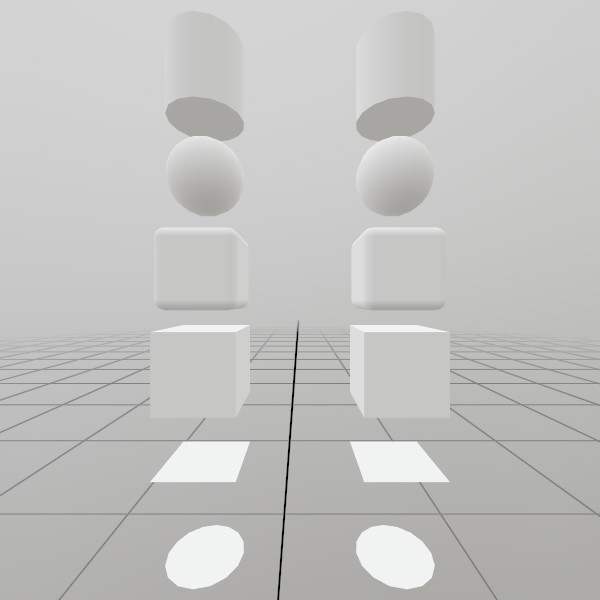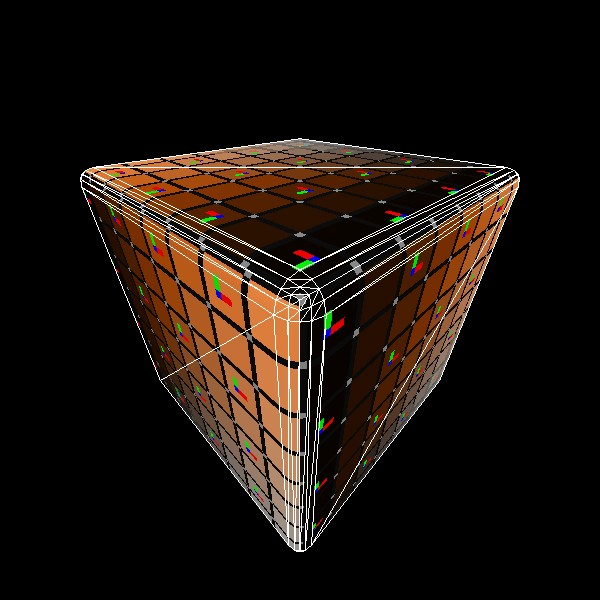# Mesh.GenerateRoundedCube

``````static Mesh GenerateRoundedCube(Vec3 dimensions, float edgeRadius, int subdivisions)
``````

Generates a cube mesh with rounded corners, pre-sized to the given dimensions. UV coordinates are 0,0 -> 1,1 on each face, meeting at the middle of the rounded corners.

NOTE: This generates a completely new Mesh asset on the GPU, and is best done during ‘initialization’ of your app/scene.

Vec3 dimensions How large is this cube on each axis, in meters?
float edgeRadius Radius of the corner rounding, in meters.
int subdivisions How many subdivisions should be used for creating the corners? A larger value results in smoother corners, but can decrease performance.
RETURNS: Mesh A cube mesh with rounded corners, pre-sized to the given dimensions.

## Examples

### Generating a Mesh and ModelHere’s a quick example of generating a mesh! You can store it in just a Mesh, or you can attach it to a Model for easier rendering later on.

``````// Do this in your initialization
Mesh  roundedCubeMesh  = Mesh.GenerateRoundedCube(Vec3.One * 0.4f, 0.05f);
Model roundedCubeModel = Model.FromMesh(roundedCubeMesh, Default.Material);
``````

Drawing both a Mesh and a Model generated this way is reasonably simple, here’s a short example! For the Mesh, you’ll need to create your own material, we just loaded up the default Material here.

``````// Call this code every Step

Matrix roundedCubeTransform = Matrix.T(-.5f, 0, 0);
roundedCubeMesh.Draw(Default.Material, roundedCubeTransform);

roundedCubeTransform = Matrix.T(.5f, 0, 0);
roundedCubeModel.Draw(roundedCubeTransform);
``````

### UV and Face layout

Here’s a test image that illustrates how this mesh’s geometry is laid out.``````meshRoundedCube = Mesh.GenerateRoundedCube(Vec3.One, 0.05f);
``````

Found an issue with these docs, or have some additional questions? Create an Issue on Github!